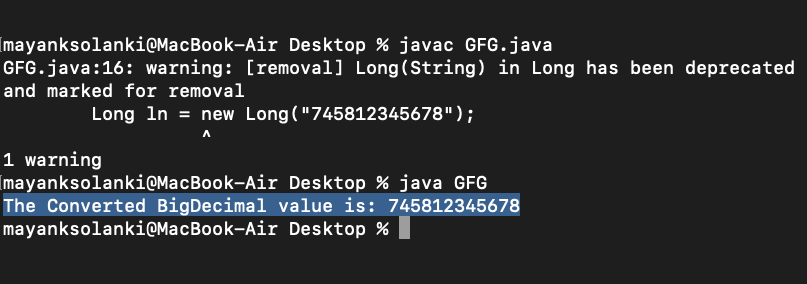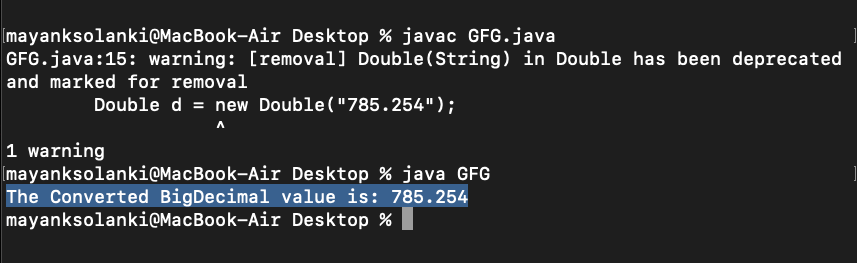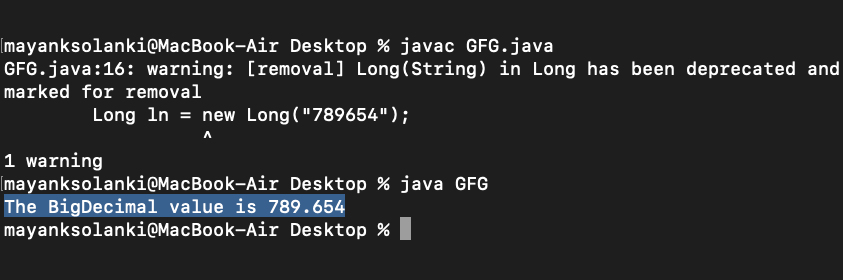# BigDecimal valueOf() Method in Java

java.math.BigDecimal.valueOf(long val) is an inbuilt method in Java that translates a long value into a BigDecimal value with a scale of zero. It allows us, the reuse of frequently used BigDecimal values and hence this “static factory method” is provided in preference to a (long) constructor. Package view is as follows:

```--> java.math Package
--> BigDecimal Class
--> valueOf() Method```

There are 3 types of variations in valueOf() method of BigDecimal class that are as depicted in syntax below via differing in parameters that are as follows:

• public static BigDecimal valueOf(long val)
• public static BigDecimal valueOf(double d)
• public static BigDecimal value(long l, int x)

### Type 1: java.math.BigDecimal.valueOf(long val)

Parameters: The method accepts a single parameter val of Long data type and refers to the value that needs to be translated into a BigDecimal value.

Return value: The method returns a BigDecimal value of Long val.

Example:

## Java

 `// Java Program to Illustrate valueOf() Method ` `// of BigDecimal Class ` `// Over long As Arguments ` ` `  `// Importing required classes ` `import` `java.math.*; ` ` `  `// Class ` `public` `class` `GFG { ` ` `  `    ``// Main driver  method ` `    ``public` `static` `void` `main(String[] args) ` `    ``{ ` ` `  `        ``// Creating and declaring long value by ` `        ``// creating object of Long class ` `        ``Long ln = ``new` `Long(``"745812345678"``); ` ` `  `        ``// Assigning above long value to BigDecimal ` `        ``BigDecimal b = BigDecimal.valueOf(ln); ` ` `  `        ``// Printing the BigDecimal value on console ` `        ``System.out.println( ` `            ``"The Converted BigDecimal value is: "` `+ b); ` `    ``} ` `}`

Output:### Type 2: java.math.BigDecimal.valueOf(double val)

The java.math.BigDecimal.valueOf(double val) is an inbuilt method in java that translates a double into a BigDecimal, using the double’s canonical string representation provided by the Double.toString(double) method.

Syntax:

`public static BigDecimal valueOf(double val)`

Parameters: The method accepts a single parameter val of Double data type and refers to the value that needs to be translated into a BigDecimal value.

Return value: The method returns a BigDecimal value which is equal to or approximately equal to Double val

Example:

## Java

 `// Java Program to Illustrate valueOf() Method ` `// of BigDecimal Class ` `// Over Double type As Arguments ` ` `  `// Importing required classes ` `import` `java.math.*; ` ` `  `// Class ` `public` `class` `GFG { ` ` `  `    ``// Main driver method ` `    ``public` `static` `void` `main(String[] args) ` `    ``{ ` ` `  `        ``// Creating a Double Object ` `        ``Double d = ``new` `Double(``"785.254"``); ` ` `  `        ``/// Assigning the bigdecimal value of d to b ` `        ``BigDecimal b = BigDecimal.valueOf(d); ` ` `  `        ``// Printing BigDecimal value on console ` `        ``System.out.println( ` `            ``"The Converted BigDecimal value is: "` `+ b); ` `    ``} ` `}`

Output:### Type 3: java.math.BigDecimal.valueOf(long unscaledVal, int scale)

The java.math.BigDecimal.valueOf(long unscaledVal, int scale) is an inbuilt method in Java that is used to translate a long unscaled value and an int scale into a BigDecimal. This “static factory method” is provided in preference to a (long, int) constructor because it allows for reuse of frequently used BigDecimal values.

Syntax:

`public static BigDecimal valueOf(long unscaledVal, int scale)`

Parameters: It takes two parameters:

• unscaledVal: This is of Long data type and refers to the Unscaled value of the BigDecimal.
• scale: This is of Integer data type and refers to the Scale of the BigDecimal.

Return Value: A big decimal whose value is unscaled*10-scale.

Example:

## Java

 `// Java Program to Illustrate valueOf() Method ` `// of BigDecimal Class ` `// Over (long, int) As Arguments ` ` `  `// Importing required classes ` `import` `java.math.*; ` ` `  `// Class ` `public` `class` `GFG { ` ` `  `    ``// Main driver method ` `    ``public` `static` `void` `main(String[] args) { ` `       `  `        ``// Creating a Long Object by ` `        ``// creating object of Long class type ` `        ``Long ln = ``new` `Long(``"789654"``); ` ` `  `        ``// Assigning the bigdecimal value to ` `        ``// BigDecimal with scale 3 ` `        ``BigDecimal b = BigDecimal.valueOf(ln, ``3``); ` ` `  `        ``// Printing BigDecimal value on console ` `        ``System.out.println(``"The BigDecimal value is "` `+ b); ` `    ``} ` `}`

Output:Whether you're preparing for your first job interview or aiming to upskill in this ever-evolving tech landscape, GeeksforGeeks Courses are your key to success. We provide top-quality content at affordable prices, all geared towards accelerating your growth in a time-bound manner. Join the millions we've already empowered, and we're here to do the same for you. Don't miss out - check it out now!

Previous
Next